Subscribe to our newsletter to receive the latest news and events from TWI:

# Comparison of J Equations for SENT Specimens

Philippa Moore and Emily Hutchison
TWI Ltd, Granta Park Great Abington, Cambridge, CB21 6AL, UK

Paper presented at 21st European Conference on Fracture, ECF21, 20-24 June 2016, Catania, Italy

## Abstract

A test standard for single edge notched tension (SENT) fracture toughness testing was issued in 2014 by British Standards as BS 8571:2014. Prior to BS 8571, most guidance on SENT test methodology was taken from DNV RP F108:2006 for installation of girth welds under high strain, which is still commonly cited by pipeline design and fabrication standards such as DNV OS F101:2013 for subsea pipelines. The intention of BS 8571 was to maintain some continuity with DNV RP F108 to allow users time to adopt the new Standard, and therefore BS 8571 included the DNV specimen design and J formulae, but also offered a different J approach based on work by Shen & colleagues (2009) from CanmetMaterials, for specimen designs currently beyond those permitted in DNV RP F108 but included in BS 8571. However, recent independent research by Zhu & McGaughy (2015) has identified an error in the K solutions adopted from DNV RP F108 into BS 8571, and therefore, there is a need to review the various equations to calculate J for SENT specimens to ensure that the optimum approach is employed in BS 8571, both in terms of accuracy and ease of application. A review of different published solutions for the elastic K solutions, and plastic eta factor solutions, which comprise parts of the calculation of J has been carried out, to compare the range of possible solutions in relation to those currently used in BS 8571:2014. Furthermore numerical modelling of crack tearing resistance curves was undertaken to compare the two different methods currently given in BS 8571 to determine which would provide the best single approach appropriate for all SENT specimens tested to BS 8571. The analyses determined that the ‘Canmet’ solutions should be used for a single equation for J to cover specimens with W/B ratios from 0.5 to 2 in future versions of BS 8571. This change will improve the accuracy of the determination of J from SENT specimens, with a simpler method, but little difference to the values of J for typical specimens which have been tested using BS 8571 to date.

© 2016 The Authors. Published by Elsevier B.V. Peer-review under responsibility of the Scientific Committee of ECF21.

## 1. Introduction

A test standard for SENT testing was issued in 2014 by British Standards as BS 8571:2014 , intended to be a general test standard, equivalent to established single edge notched bend (SENB) test standards. This means that fracture toughness in terms of crack tip opening displacement (CTOD) as well as J-integral can be calculated, that single-point fracture toughness as well as tearing resistance curves (R-curves) can be determined, and that validity limits are given to confirm whether the test has been carried out satisfactorily. Prior to BS 8571, most guidance on SENT test methodology was taken from DNV RP F108:2006 for installation of girth welds under high strain, which provides a relatively limited set of test conditions; only multiple-specimen J R-curves in specimens with width-to-thickness (W/B) ratio up to 1. Other SENT testing methods from literature describe alternative methods to determine J using unloading compliance techniques in SENT specimens, most notably Cravero and Ruggieri (2007), and Shen et al. (2009). The intention of BS 8571 was to maintain some continuity with DNV RP F108 to allow users some time to adopt the new Standard, and therefore included the DNV specimen design and J formulae. An alternative J equation was offered for specimens with W/B ratios between 1 and 2.

Zhu and McGaughy (2015) recently identified an error in the stress intensity factor (K) solutions adopted from DNV RP F108 into BS 8571 to evaluate the elastic component of J for clamped specimens. Their paper evaluated the K solutions in BS 8571 through a comparison with numerical calculations of K obtained using finite element analysis for clamped single edge notched tension (SENT) specimens. Their recommendation was for the BSI committee to consider removing the DNV K solutions from the standard, and having just one method for determining J for all specimen designs. In addition, there have been a number of papers presenting alternative eta factor solutions, used for the determination of the plastic component of J, which also deserve consideration as potential alternatives to those currently adopted in BS 8571.

### 1.1. Nomenclature

 a0 initial crack length (comprising the machined notch and fatigue pre crack length), (mm) b0 Initial specimen ligament ahead of the notch equal to W-a0 (mm) n Strain hardening exponent B Specimen thickness perpendicular to the width (mm). BN Net section specimen thickness after side grooving (mm) E Modulus of elasticity (N/mm2) E’ Longitudinal elastic modulus in plane strain, equal to E/(1-ν2), (N/mm2) H Length of the SENT specimen between the grips (mm) Jel Elastic component of J, (kJ/m2 or N/mm) Jpl Plastic component of J, (kJ/m2 or N/mm) K Stress intensity factor (see section 2.3), (N/mm3/2) My Ratio of the weld metal yield strength to the parent metal yield strength P Applied load, N Up Area under the plastic part of the load versus crack mouth opening displacement (CMOD) curve, (Nmm) W Specimen width, measured in the direction of the notch, (mm) η Dimensionless function of geometry (see section 2.4) ν Poisson’s ratio

## 2. Equations for J

### 2.1. General J equations

The general form for equations to calculate J is given in equation 1, based on a sum of the elastic and plastic components of J. The elastic component is based upon the stress intensity factor, K, while the plastic part is calculated from the area under the load-displacement curve generated during the fracture toughness test combined with a geometrical factor known as the eta (η) factor. BS 8571 adopted two approaches to determine J for clamped specimens. The first one is currently in DNV RP F108 (2006) and is based on an analytical K solution originally obtained by Ahmad et al. (1991), and an eta factor solution developed specifically for the DNV procedure. This is the default equation for calculating J given in BS 8571 for clamped SENT specimens with W/B ratios up to 1, and in this paper, this approach to determine J is known as the ‘DNV equation’. An alternative equation for J is given in BS 8571 for clamped SENT specimens with W/B ratios greater than 1 (outside the validity of the original DNV equation). It is of a very similar basic form to the DNV equation, but defines different equations for K and for η than DNV, and includes the net thickness after side-grooving (where used) in place of B. This equation is based on numerical solutions for K and eta from work by Shen et al. (2009) at CanmetMaterials, but simplified for a single crack length rather than a growing crack, so that it can be used for single point results as an alternative to the DNV equation. In this paper, this method is referred to as ‘Canmet Static’ to identify it as the equation for a static crack rather than the more complex original form of the equation for a growing crack. The originally equation in Shen et al, (2009) includes incremental terms for J calculations based on each step of tearing that increases the crack length as the crack grows, as well as the initial crack depth, and can be used for J R-curves to account for stable crack tearing. This method is referred to as the ‘Canmet equation’ in this paper, and is considered alongside the two methods currently in BS 8571 as a potential method for inclusion in future versions of BS 8571 for unloading compliance R-curve tests. These are the three principal J equations which are compared in this work, although solutions from other sources are discussed.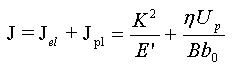(1)

### 2.2. Stress intensity factor (K) equations

For clamped specimens, the DNV equation for K is given by equation 2, where f1, f2 and ξ3 are complex functions including polynomial equations in terms of a0/W and Heaviside functions. The Canmet equation for K has the same first term (inside the square brackets) as DNV, but a simpler polynomial function of a0/W valid for 0.05 ≤ a0/W ≤ 0.95. Cravero & Ruggieri (2007) also offer a formula for K, which is similar to that of Canmet but with different coefficients for the polynomial function of (a0/W), however their first square bracketed term is simply P/B√W. Zhu & McGaughy compared these three equations for K, and found very little difference between the Canmet (Shen et al. (2009)) and the Cravero & Ruggieri (2007) equations for a/W ratios from 0.1 up to 0.9. The Ahmad et al. (1991) solution was very different to the others above a/W of 0.6, and Zhu & McGaughy (2015) report that this is due to an error in the original calculations by Ahmad et al (1991), which had not been corrected when the solution was used in DNV RP F108:2006 or BS 8571:2014. Zhu & McGaughy (2015) determine that there is less than 1% inaccuracy in the Canmet K formula for a0/W between 0.05 and 0.77 (i.e. more than covering the range permitted in BS 8571).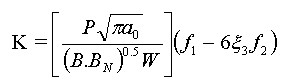(2)

### 2.3. Eta (η) factor equations

Eta (η) is a non-dimensional parameter which describes the effect of the plastic strain energy of the applied J integral, and is used in the calculation of the plastic component of J (Carvalho and Ruggieri, 2010). The precise details of the equations to calculate η depend on whether it is based on the load-line displacement (LLD) or the crack mouth opening displacement (CMOD) measurements, and on whether it is for pin-loaded or clamped SENT tests. There have been a significant number of sources of different η factors for SENTs in recent years, and most references give a polynomial equation as a function of a/W based on functions fitted to numerical analyses of SENT specimens. In this paper, only the solutions for clamped specimens based on CMOD with specimen length H equal to ten times the width W which have been compared. The solution in DNV RP F108 (2006), equation 3, is based on equations for a stationary crack in parent material, but includes an additional 0.85 factor to allow for effects of weld strength mismatch and strain hardening (Pisarski, 2010). Canmet (Shen et al., 2009) provide a simpler equation, but with a larger number of polynomial orders up to ten, and is considered to be independent of strain hardening. More recent η factor solutions have been published by Ruggieri (2012), which is of a similar form to Canmet but with different coefficients for parent material with strain hardening coefficient (n) of 5 (typical of high strength steel), 10 (which might reflect a structural steel), and 20 (closer to the tensile behaviour of stainless steels). A general form of all these is given in equation 4. These three different approaches were compared by Wang et al (2015), who also offer their own (more complex) approach, where the main coefficients of a0/W are defined as θiχi, the respective components of which incorporate, the strain hardening coefficient, n, and the BN/W ratio of the specimen, as variables. These different parent material eta functions are plotted in Fig. 1a. Specific η solutions for weld specimens have also been published by Paredes & Ruggieri (2012), for different levels of weld strength mismatch ratio, My, up to 1.5. An alternative eta factor equation for weld specimens is offered by Moreira & Donato (2010), based on the weld width, 2h, as well as My, in a twenty-term equation. These eta functions for welds are plotted in Fig.  1b.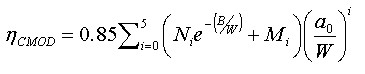(3)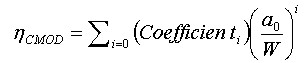(4)

When these eta factor functions are plotted against a/W ratio in Fig. 1 it is possible to compare them. The DNV solution (even accounting for the 0.85 factor) is higher than almost all of the others. The Canmet solution is close to other solutions for low levels of strain hardening and/or weld strength overmatch. The Paredes weld equations give significantly lower eta factors than Moreira and Donato, and seem conservative compared to the other solutions. The Wang solutions are close to the Canmet solution within the current a/W range in BS 8571 of 0.2 to 0.5, but diverge beyond this. All the solutions presented here have been individually validated against their own numerical models, so it is not possible to identify from this comparison which is ‘best’ in terms of accuracy. However, the Canmet formula is not an outlier and captures the trend of many of the other solutions, so is a reasonable choice of general eta factor solution for SENT tests. The assumption about eta might need to be further reviewed when testing very high weld strength overmatching (My > 1.3) or for very high strain hardening materials (n>10)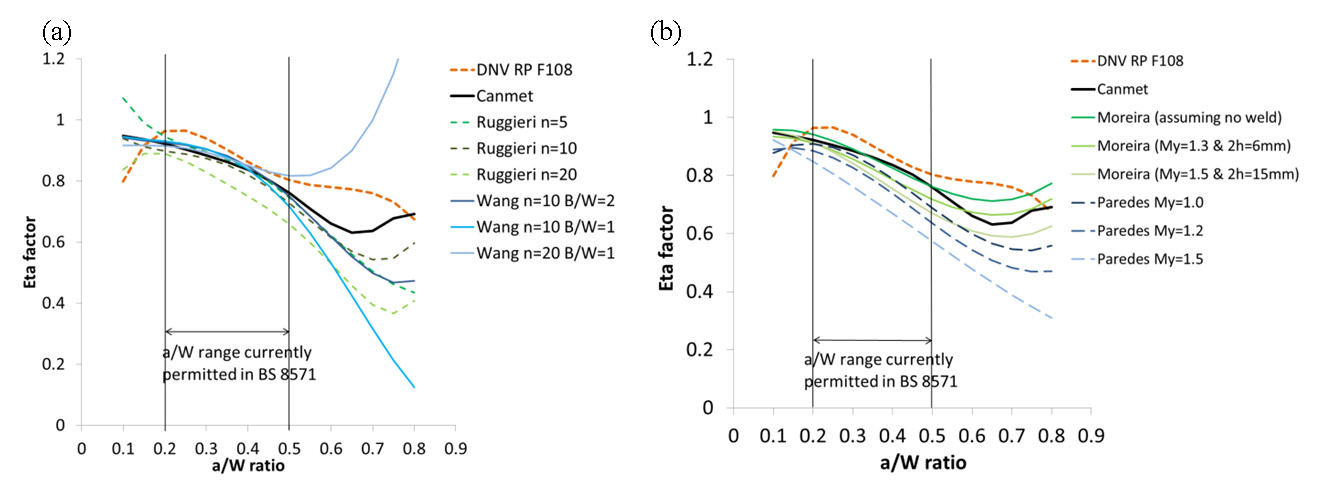Fig. 1. (a) Comparison of plastic eta factors from literature for parent materials with different strain hardening coefficients (n). (b) Comparison of plastic eta factors from literature for welds of different strength mismatch (My) and weld width (2h), alongside the DNV and Canmet formulae.

## 3. Comparison of J Equations

### 3.1. SENT test data

The comparison of the different methods to determine J was initially made by comparing results from experimental SENT tests with single value results, and comparing the J that would be determined using the two alternatives in BS 8571. The experimental data presented here were taken from several programmes of SENT testing work at TWI. All the data is from specimens tested using the clamped test configuration (Fig. 2). The test data for the single point comparison has been collated from:

1. Specimens from a girth weld in 22in OD and 15.9mm WT pipe with weld metal that even-matched the parent strength of 550MPa. The specimens had a/W ratio of 0.4 and were tested at -60°C to -85°C.
2. Specimens from the fusion line of a 14.3in OD and 24mm WT GMAW welded pipe, with parent and weld metal yield strengths of 506MPa and 690MPa. Specimens with a/W of 0.35, 0.43 and 0.51 were tested at -80°C.
3. Weld metal specimens of 20mm thick, GMAW welded X70 pipe. The parent and weld metal had yield strengths of 478MPa and 604MPa. The specimens with a/W ratio of 0.4, were tested at -20°C and -80°C.
4. Parent metal specimens from 26mm thick SA543 Grade B high strength steel plate with 0.2% proof strength of 850MPa. Specimens were prepared to a nominal a/W ratio of 0.5 and tested between -40°C and -140°C.
5. Parent metal specimens from 26mm thick SA302 Grade C structural steel plate with 0.2% proof strength of 640MPa. Specimens were prepared to a nominal a/W ratio of 0.5 and tested between +60°C and -80°C.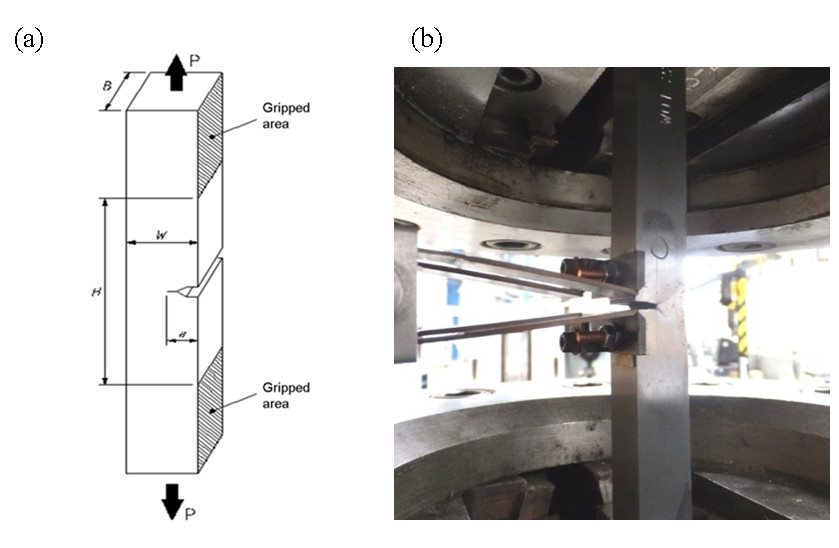Fig. 2. (a) SENT test specimen with a BxB square section design, (b) an SENT test specimen instrumented with a double clip gauge.

The test data for the comparison of the R-curves, based on numerical modelling, have been given identification numbers between 1 and 6; they have been collated from:

1. The same weld material as listed as c) above, but specimens tested room temperature to generate a multiple-specimen R-curve. This is identified as ‘Mat 3’ in the numerical modelling.
2. Specimens notched to a/W of 0.4 into the weld metal of a girth weld in 22in OD and 19.1mm WT pipe. The parent and weld metal yield strengths were 570MPa and 543MPa. Specimens with side grooves from 0% up to 10% each side generated unloading-compliance J R-curves. This is identified as ‘Mat 6’ in the numerical modelling.
3. The same weld material as listed g) above was used to produce equivalent specimens notched into the parent metal to determine comparison R-curves. This is identified as ‘Mat 1’ in the numerical modelling.
4. Specimens from 18mm thick seamless pipe with yield strength of 510MPa were notched to a nominal a/W of 0.3, and generated a multiple specimen J R-curve. This is identified as ‘Mat 5’ in the numerical modelling.
5. The parent pipe listed as i), was girth welded to give weld metal with yield strength of 627MPa. Specimens with a nominal a/W of 0.34 gave a multiple specimen J R-curve. This is identified as ‘Mat 4’.
6. Parent metal SENT specimen from 23mm thick pipe was notched to an a/W ratio of 0.5, and gave a room temperature unloading compliance J R-curve. This is identified as ‘Mat 2’ in the numerical modelling.
The true stress-strain curves from these latter six materials, and the tearing resistance curves as power law curve fits to experimental data from the materials, were used for various numerical model cases as given in Table 1.

### 3.2. Numerical models

To assess the significance of all three J equations on SENT R-curves, a set of numerical models was developed based on several different materials properties, and three different notch depth to width (a/W) ratios. Experimental R-curves were used to calculate the level of crack driving force, J, required for each tearing increment in the model. A Young’s modulus of 207GPa and Poisson’s ratio of 0.3 were assumed in the models. All models were generated using version 6.13-1 of the pre-processing finite element software Abaqus/CAE and the analyses solved using version 6.13-1 of Abaqus/Standard. Stable tearing was simulated using the nodal release method with a known J-R curve. The SENT specimen with the initial crack depth, a0, is loaded until it reaches the crack driving force (J1) corresponding to the first increment of crack tearing (Δa1). At this point, the nodes ahead of the crack tip corresponding to the tearing increment, Δa1, are released to simulate crack tearing, while no further load is applied. This process is repeated over a number of tearing increments to simulate stable ductile tearing.

A BxB SENT geometry was modelled, with B=W=14mm. The specimens were modelled using 2D plane strain elements with half-symmetry on the ligament of the crack plane. The mesh for the model comprised of quadratic, quadrilateral plane strain elements (type CPE8R in Abaqus), with approximately 3,000 elements per model. A fine, focussed mesh was employed near the crack tip, where the largest stress gradient was expected. The focused mesh had multiple rings of elements around the crack tip to create paths for the calculation of J using contour integrals. A coarser mesh was employed away from the crack tip to reduce computation time. After the first calculation step, when the crack driving force was equal to the J for 0.2mm of tearing, the first tearing boundary condition was deactivated. This process was repeated, applying the load corresponding to a given crack driving force and then calculating each tearing increment iteratively for all tearing increments. The loading of the specimen and deactivation of boundary conditions were applied in separate steps in order to aid convergence. Tearing increments of 0.1mm in size were used with approximately 10 increments in each model.

For each model case in Table 1, the area under the load against CMOD curve was output from the FEA, and J was calculated using the three different equations described in section 2.1. These values of J were compared with J calculated using the contour integral method from the FEA. The experimental input data was based on J calculated from BS 8571 (and therefore the DNV equation) so the DNV solution would almost exactly match the experimental R-curve, but this method allows the other J equations to be compared as equivalent R-curves. All the models were geometrically the same apart from the a/W ratio, where cases of 0.3, 0.4 and 0.5 were assessed, and the six different materials were investigated.

Table 1. Cases studied for crack tearing, for different materials and R-curves, showing the curve fitting parameters for the experimental R-curves.

 Case Material a/W J R-curve J=m+lΔax m l x 1 1 0.4 1 -50786 52057 0.01 2 1 0.3 1 -50786 52057 0.01 3 1 0.5 1 -50786 52057 0.01 4 2 0.4 2 -1640 2747 0.25 5 2 0.4 3 -570 1523 0.36 6 3 0.4 4 63 600 0.79 7 4 0.4 5 0 883.18 0.62 8 5 0.4 6 0 1169.2 0.71 9 6 0.4 7 -1460 2489 0.17 10 6 0.4 8 -43830 44775 0.01 11 6 0.4 9 -1642 2623 0.18

## 3. Results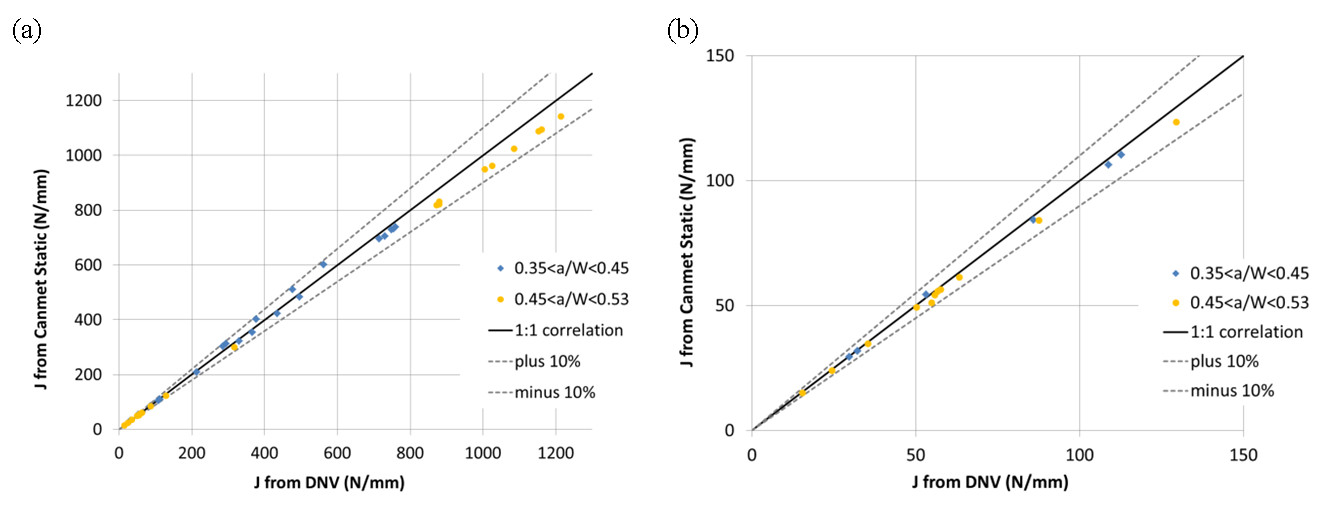Fig. 3. (a) Full set of data showing comparison of experimental SENT single point results calculated using DNV and Canmet Static equations. Also shown are the 1:1 correlation line and ±10% error lines , (b) selection from (a) but just showing data at low values of J.

The collection of single-point SENT tests data from recent TWI projects is plotted in Fig. 3, with the J calculated using the DNV equation correlated against the J from the Canmet static equation. At low values of J the comparison is dominated by the elastic component of J (Fig. 4b), whereas at high values of J it is the plastic component which has a dominant influence (Fig. 4a). All of the data falls within the 10% error bands around the 1:1 correlation. This means that there is little significant difference in the experimental values of J calculated using either of these methods. The data is shown separately for SENT tests with a/W ratios of 0.35 to 0.45, and for a/W ratio of 0.45 to 0.51. The deeper notch depth shows a slightly lower Canmet Static J compared to DNV at high values of J. The shallower-notched specimens correlate around 1:1 but with more scatter; a/W ratio has less effect for J < 150N/mm.

The three J equations (DNV, Canmet and Canmet Static) were compared for model case 1 and were within 2% of the results from FEA (i.e. the input J-R curve data). The J equations for a/W ratios of 0.3 and 0.5 (cases 2 and 3) deviated from the FEA results by more than for case 1, but all results were still within 10.5% of the FEA value for J. For a/W of 0.3, the J equations all overestimated the J-R curve compared with FEA. For a/W of 0.5, the J equations all underestimated the value of J compared with FEA. The J-R curves calculated using the three equations and the J-R curve calculated by FEA for cases 4 to 11 (Table 3) showed very similar trends as case 1. The results show that for the same specimen geometry with a/W of 0.4 there is very little difference between the J calculation methods with these different materials behaviour, with a maximum of 7.5% difference between any of the calculations and the FEA results. All of the different J equations tend to overestimate J slightly compared with FEA.

## 5. Discussion

The desire to review the J equations used in BS 8571 is partly based on the desire to simplify the approach, and using one equation for J for all W/B dimensions would be an improvement. Currently when BxB specimens are being tested, they fall at the cut-off between the two J equations in BS 8571, giving the anomalous situation that slight differences in machining tolerances can cause some specimens within a set to have W/B just less than 1 and be analysed to a different equation to specimens with W/B slightly greater than 1. This does not seem an acceptable situation for a test standard. However, any new J equations for SENT testing may take time to implement within established testing labs due to the software alterations required. Any intention to alter the equations in BS 8571 will be based on the evidence provided to the BSI committee and their collective decision.

The results that are presented here were evaluated for accuracy, alongside other considerations which influence the ultimate choice of the J approach. Of course, whichever method is implemented in BS 8571 must provide an accurate value of J, and the results of this work contribute to that evaluation. The DNV method nonetheless also provides continuity with the SENT approach generally used by industry over the past decade. However, the analytical K solution from DNV is correct only for crack length to specimen width ratio a/W≤0.6, but incorrect for a/W>0.6. Zhu and McGaughy (2015) found that Ahmad et al. (1991) made mistakes in their mathematical derivation of the K solution for deep cracks of a/W>0.6. Although this a/W ratio is outside the range currently permitted in BS 8571, this error, coupled with the complex Heaviside functions which must be processed in order to determine J, means that a simpler, more accurate alternative to the DNV equation would be a practical benefit. The different K functions vary only with a/W and are not dependent on the specimen width or thickness, implying that a common formula for all SENT specimen designs should be appropriate.

When a comparison of the two J equations (DNV and Canmet) is made based on a set of experimental test data, there was very little difference between the results of the two, suggesting that for the common SENT applications to date, the errors in the DNV method would not have been significant. The comparison of single point values of J show that there is less than 10% difference between the J determined from the DNV equation and the Canmet static equation. This means that should the J equation in BS 8571 be altered to solely include those from Canmet, the difference in the single point values of J being determined as a consequence would be negligible.

The J equations for DNV, Canmet and Canmet Static all agree to within less than 10.5% of the FEA values for the J-R curves up to the allowable level of crack tearing of 20% of the specimen ligament according to BS 8571 (2014). This is true over a range of geometry and material properties. This suggests that all of the equations can be considered valid for calculating the J-R curve from experimental data. The Canmet static equation is a simpler alternative to DNV, and has the advantage that it also has the growing crack form of the method which may be implemented equivalently for single specimen R-curve methods, providing a common approach to J determination for single value tests and R-curves.

## 6. Conclusions & Recommendations

The recommendation from this work is that in future versions of BS 8571 there should be a single equation for J to cover specimens with W/B ratios from 0.5 to 2 based on the Canmet static equation which is already partially implemented in that standard.

This change will improve the accuracy of the determination of J from SENT specimens, with a simpler method, but little difference to the values of J for typical specimens which have been tested using BS 8571 to date.

The use of the full Canmet equation would be a suitable addition, possibly within a new annex in BS 8571 for unloading compliance J R-curve tests.

Any future changes made to the J equations in BS 8571 might take some time to become fully implemented through test houses worldwide, which have become used to using the DNV formulae.

These results and recommendations will be put to the British Standards committee responsible for BS 8571 for consideration for inclusion into future versions of that standard.

## 7. Acknowledgements

The work presented here was partly carried out as part of a joint-industry project funded by Subsea7, Saipem, BP Ltd and TWI Ltd. Thanks to the sponsors of this work for their support throughout the project, and their permission to publish. Thanks also to Phillip Cossey at TWI for carrying out the experimental SENT tests presented here.

## 8. References

1. Ahmad, J., Papaspyropoulos, V., Hopper, A. T., 1991. Elastic-Plastic Analysis of Edge-Notched Panels Subjected to Fixed Grip Loading. Engineering Fracture Mechanics, Vol. 38, pp. 283-294.
BS 8571, 2014 ‘Method of test for determination of fracture toughness in metallic materials using single edge notched tension (SENT) specimens’, British Standards Institution, December 2014.
2. Carvalho, H. S. S., Ruggieri, C., 2010. Significance of the plastic eta factor in J estimation procedures for tensile SE(T) fracture specimens. PVP2010 ASME Pressure Vessels and Piping Conference, Bellevue, Washington, USA..
3. DNV ‘Fracture control for pipeline installation methods introducing plastic strain’, Det Norske Veritas Recommended Practice DNV-RP-F108, 2006.
4. Cravero, S., Ruggieri, C., 2007. Estimation procedure of J-resistance curves for SE(T) fracture specimens using unloading compliance. Engineering Fracture Mechanics Vol. 74, pp 2735-2757.
5. Moreira, F., Donato, G., 2010. Estimation procedures for J and CTOD fracture parameters experimentally evaluation using homogeneous and mismatched clamped SE(T) specimens. ASME Pressure Vessels and Piping Conference PVP2010, Bellevue, Washington, USA.
6. Paredes, M., Ruggieri, C., 2012. Further results in J and CTOD estimation procedures for SE(T) fracture specimens – Part II: Weld centreline cracks. Engineering Fracture Mechanics, Vol. 89, pp 24-39.
7. Pisarski, H., 2010. Determination of pipe girth weld fracture toughness using SENT specimens. 8th International Pipeline Conference IPC2010, Calgary, Canada.
8. Ruggieri, C., 2012. Further results in J and CTOD estimation procedures for SE(T) fracture specimens – Part I: Homogeneous materials. Engineering Fracture Mechanics, Vol. 79, pp 245-265.
9. Shen, G., Gianetto, J., Tyson, W., 2009. Measurement of J-R Curves using single specimen technique on clamped SE(T) specimens. 19th International Offshore and Polar Engineering Conference ISOPE2009, Osaka, Japan.
10. Wang, E., De Waele, W., Hertelé, S., 2015. A complementary ηpl approach in J and CTOD estimations for clamped SENT specimens. Engineering Fracture Mechanics, Vol. 147, pp 36-54.
11. Zhu, X.-K., McGaughy, T., 2015. Corrected Stress Intensity Factor for BS 8571 Single Edge Notched Tension (SENT) Specimens. 25th International Offshore and Polar Engineering Conference ISOPE2015, Kona, Hawaii, USA.